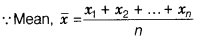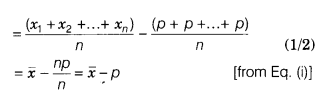# The mean of n observations x1, x2,. ..., xn is x. If each observation is decreased by p

The mean of n observations x1, x2,. …, xn is x. If each observation is decreased by p, then show that the mean of the new observations is (x - p).If each observation is decreased by p, then new observations become x1-p,x2-p,…xn-p